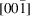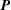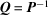International Tables for Crystallography (2006). Vol. A, ch. 5.1, pp. 78-85https://doi.org/10.1107/97809553602060000510

## Contents

• 5.1. Transformations of the coordinate system (unit-cell transformations)  (pp. 78-85)
• 5.1.1. Introduction  (p. 78) | html | pdf |
• 5.1.2. Matrix notation  (p. 78) | html | pdf |
• 5.1.3. General transformation  (pp. 78-85) | html | pdf |
• Figures
• Fig. 5.1.3.1. General affine transformation  (p. 79) | html | pdf |
• Fig. 5.1.3.2. Monoclinic centred lattice, projected along the unique axis  (p. 83) | html | pdf |
• Fig. 5.1.3.3. Body-centred cell I with a , b , c and a corresponding primitive cell P with a ′, b ′, c ′  (p. 83) | html | pdf |
• Fig. 5.1.3.4. Face-centred cell F with a , b , c and a corresponding primitive cell P with a ′, b ′, c ′  (p. 83) | html | pdf |
• Fig. 5.1.3.5. Tetragonal lattices, projected along(p. 84) | html | pdf |
• Fig. 5.1.3.6. Unit cells in the rhombohedral lattice  (p. 84) | html | pdf |
• Fig. 5.1.3.7. Hexagonal lattice projected along(p. 84) | html | pdf |
• Fig. 5.1.3.8. Hexagonal lattice projected along(p. 85) | html | pdf |
• Fig. 5.1.3.9. Rhombohedral lattice with a triple hexagonal unit cell a , b , c in obverse setting  (p. 85) | html | pdf |
• Fig. 5.1.3.10. Rhombohedral lattice with primitive rhombohedral cell a , b , c and the three centred monoclinic cells  (p. 85) | html | pdf |
• Tables
• Table 5.1.3.1. Selected 3 × 3 transformation matricesand(pp. 80-83) | html | pdf |